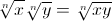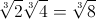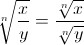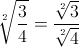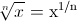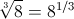# SAT Test Prep #2: Power Rules of Exponents

Filed under Algebra, Difficulty: Easy, SAT, Test Prep.

While you will be able to use a graphing calculator on the SAT, sometimes it is faster to have mathematical rules memorized beforehand so you can quickly solve problems without trying to find the answer by trial and error with your calculator. One type of problem you may encounter on the SAT involves exponents and powers ( for example, (32)4 ).

In this post, we present some easy to remember rules for solving exponents and radicals. By memorizing these rules for simplifying any exponent or radical problem you encounter, you’ll save yourself valuable time while taking the SAT. Keep reading to learn how to add, subtract, multiply and divide exponents and radicals.

### Power Rules

 Format Example xm × xn = x(m+n) 42 × 43 = 4(2+3) = 45 xm ÷ xn = x(m-n) 34 ÷ 32 = 3(4-2) = 32 (xm )n = x(m×n) (23 )2 = 2(3×2) = 26 (xy)n = xn × yn (2x)3 = 23 × x3 = 8x3 (x / y)n = xn ÷ yn (2 / 3)3 = 23 ÷ 33 = 8/27 (x)-n = 1/(x)n (4)-2 = 1/(4)2

 Format Example